Students can access the CBSE Sample Papers for Class 12 Physics with Solutions and marking scheme Term 2 Set 7 will help students in understanding the difficulty level of the exam.

## CBSE Sample Papers for Class 12 Physics Standard Term 2 Set 7 with Solutions

Time Allowed: 2 Hours
Maximum Marks: 40

General Instructions:

• There are 12 questions in all. All questions are compulsory.
• This question paper has three sections: Section A, Section B and Section C.
• Section A contains three questions of two marks each, Section B contains eight questions of three marks each, Section C contains one case study-based question of five marks.
• There is no overall choice. However, an internal choice has been provided in one question of two marks and two questions of three marks. You have to attempt only one of the chokes in such questions.
• You may use log tables if necessary but use of calculator is not allowed.

SECTION – A
(Section A contains 3 questions of 2 marks each.)

Question 1.
A student claims that she is going to eject electrons from a piece of metal by placing a radio transmitter antenna adjacent to the metal and sending a strong AM radio signal into the antenna. The work function of a metal is typically a few electron volts. Will this work? (2)
Work function= few electron volt
= 1.6 × 106 Hz
Energy of photon= $$\frac{h c}{\lambda}$$
= 6.6 × 10-9 eV

Ejection of electron is not possible by AM radio signal, as energy of its photon is less than the work function.

Caution
Students are often confused when photoetectrons eject from the metal. For it the energy of the photon must be more than the work function.Question 2.
How does an air bubble in water behaves as a diverging lens? (2)
If a parallel beam of light is incident on an air bubble in water, then the refracted rays diverge from each other.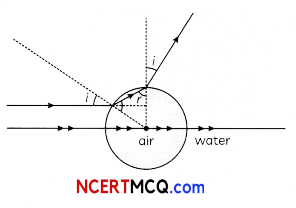Question 3.
Write two drawbacks of Rutherford’s atomic model.
OR
Write main use of the:
(A) Solar Cell
(B) Photodiode (2)
(A) It does not explain the stability of atom.

(B) In Rutherford’s model, an electron can revolve in orbits of all possible radii. So, it should emit a continuous spectrum. But an atom like hydrogen always emits a discrete line spectrum.
OR
(A) A solar cell is a junction diode which converts solar energy to electrical energy. The generation of voltage is due to constant bombardment or light photons.

(B) In demodulation and direction of optical signals, in light operated switches and an electronic counter, photodiodes are used.

Related Theory
Photodiodes are preferably operated in reverse bias condition. Even fractional increase in majority charge carriers is much less than the fractional change increases in minority charge carriers. The fractional change due to the photo effects on the minority carriers dominated reverse bias current is more easily measurable than the fractional change in the majority charge carriers dominated forward bias current.SECTION – B
(Section B consists of 8 questions of 3 marks each.)

Question 4.
What is a hole? Give their important characteristics. (3)
Vacancy or absence of electron in the bond of a covaiently bonded crystal are called holes. Whenever an electron is removed from the completely filled valence band of a semiconductor, a vacancy is left Behind in the valence band. A hole is not a physical entity but just a convenient way for describing charge motion.

(A) A hole just a vacancy created by removal of electron from a covalent bond of a semiconductor.
(B) It has the same mass as the removed electron.
(C) It is associated with the positive charge of magnitude e.

Question 5.
Light from a point source in air falls on a convex spherical glass surface of refractive index 1.5 and radius of curvature 20 cm. The distance of light source from the glass surface is 100 cm. At what position is the image formed? (3)
Given, refractive index,
n2 = 1.5,
n = 1 (air)
R = 20 cm
Object distance,
u = – 100 cm
To find, Image distance, v
$$\frac{n_{2}}{v}-\frac{n_{1}}{u}$$ = $$\frac{n_{2}-n_{1}}{\mathrm{R}}$$
$$\frac{1.5}{v}+\frac{1}{(100)}$$ = $$\frac{1.5-1}{20}=\frac{1}{40}$$
$$\frac{1.5}{v}$$ = $$\frac{1}{40}-\frac{1}{100}$$
= $$\frac{5-2}{200}=\frac{3}{200}$$
v = $$\frac{200}{3}$$
= 100 cm
The image is formed at 100 cm in denser medium.

Question 6.
(A) What is the relation between the
intensity and amplitude of wave?
(B) Two coherent light beams of intensities I and 41 are superposed. Find the maximum and minimum possible intensities in the resulting beam? (3)
(A) Intensity ∝ (Amplitude)2
(B) The net intensity due to interference of two beams of intensities I1 I2 with a phase difference of Φ) is:
Inet = I1 + I2+ 2I1I2 cos Φ
Thus, Inetmax occurs for cos Φ = 1
= Inetmax = ($$\left(\sqrt{I_{1}}+\sqrt{I_{2}}\right)$$)2 = 9I
Similarly,Inetmin inetmin occurs for cos = -1
Inetmin = ($$\left(\sqrt{I_{1}}+\sqrt{I_{2}}\right)$$)2Question 7.
In a Geiger-Marsden experiment, calculate the distance of closest approach to the nucleus of Z = 80, when α-particle of 8 MeV energy impinges on it before it comes momentarily to rest and reverses its direction. How will the distance of closest approach be affected when the kinetic energy of the α-particle is doubled?.
OR
What do you mean by coherence? How are coherent sources produced in laboratory? (3)
Let ro be the centre to centre distance between the alpha-particle and nucleus.
Given Z = 80 Ek = 8MeV= 2.88 × 10-14 m
= 28.8 fm
Since, ro ∝ $$\frac{1}{E_{k}}$$
So when kinetic energy is doubled the distance of closest approach ro is halved.
OR
Coherence is an ideal property of waves that enables stationary interference.

Methods to produce them:
(A) Division of wavefront: Wavefront from a point source of light illuminated by an ordinary source of light can be divided into two parts by allowing it to fall on a pair of slits.
(B) Division of amplitude: Light from an ordinary source may be divided into two or more beams by partial reflection. This way the amplitude of the incident beam is divided.

Question 8.
(A) Under what conditions, total internal
reflection occurs?
(B) State, with the help of a ray diagram, the working principle of optical fibres. Write one important use of optical fibres. (3)
(A) (i) Light must go from denser to rarer medium.
(ii) The angle of incidence in the denser medium must be greater than the critical angle of the pair of media.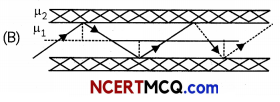Optical fibre works on principle of total internal reflection. When angle of incidence is greater than critical angle then incident rays are totally reflected back in same media.
When, θi > θc , Total internal reflection occurs and if θi < θc refraction occurs.
Application: Optical fibres are used for communication due to very high bandwidth of media.Question 9.
Explain briefly how V-l characteristics of a p-n junction diode obtained in:
(A) Forward bias
(B) Reverse biased
And draw shape of curves obtained. (3)
(A) In forward bias characteristics, it is observed that initially no current flows up to knee voltage and after knee voltage current increases rapidly.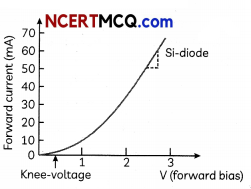(B) In reverse bias characteristics it is observed that initially very little current flows due to minority charge carriers up to breakdown voltage after which the current becomes independent of voltage.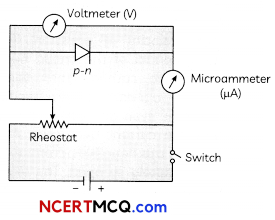Question 10.
(A) How are electromagnetic waves
produced by oscillating charges?
(B) State clearly how a microwave oven works to heat up a food item containing water molecules.
(C) Why are microwaves found useful for the radar systems in aircraft navigation? (3)
(A) If a charged particle oscillate with some frequency, produces an oscillating electric field in space, which produces an oscillating magnetic field, which in turn, is a source of electric field, and so on. Thus, oscillating electric fields and magnetic fields regenerate each other, and an electromagnetic wave propagates in the space.

(B) In microwave oven the frequency of the microwaves is selected to match the resonant frequency of water molecules so that energy from the waves get transferred efficiently to the kinetic energy of the molecules. This kinetic energy raises the temperature of any food containing water.

(C) Microwaves are short waveleangth radio waves with frequency of order of GHz. Due to short wavelength, they have high penetrating power with respect to atmosphere and less diffraction in the atmospheric layers. So, these waves are suitable for the radar systems used in aircraft navigation.Question 11.
Define the terms:
(A) ‘cut-off voltage’ and
(B) ‘threshold frequency’ in relation to the phenomenon of photoelectric effect.

Using Einstein’s photoelectric equation show how the cut-off voltage and threshold frequency for a given photosensitive material can be determined with the help of a suitable $$\frac{\text { plot }}{\text { graph }}$$
OR
In the case of plastic lens, the thickness of the lens increased in comparison of the glass lens. Justify the statement. (3)
When light of suitable frequency is incident on a metal surface, electrons are ejected from the metal. This phenomenon is called the photoelectric effect.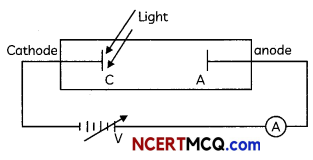(A) The cathode is illuminated with light of some fixed frequency v and fixed intensity li. A small photoelectric current is observed due to few electrons that reach anode just because they have sufficiently large velocity of emission. If we make the potential of the anode negative with respect to cathode then the electrons emitted by cathode are repelled. Some electrons even go back to the cathode so that the current decreases. At a certain value of this negative potential, the current is completely stopped. The least value of this anode potential which just stops the photocurrent is called cut off potential or stopping potential.

(B) For a given material, there is a certain minimum frequency that if the incident radiation has a frequency below this threshold, no photoelectric emission will take place, howsoever intense the radiation may be falling. This minimum frequency is called threshold frequency.
According to Einstein’s photoelectric equation, maximum K.E. is given as
K.Emax = $$\frac{h c}{\lambda-\phi}$$
= hv – Φ
Where λ, is wavelength of light and v is corresponding frequency and Φ is work function. We expose a material to lights of various frequencies and thus photoelectric current is observed and cut off potential needed to reduce this current to zero is noted. A graph is plotted and that is straight line.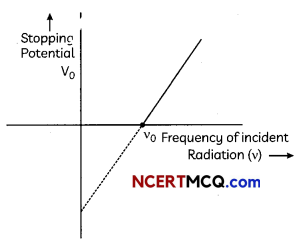According to Einstein’s phottoelectric equation
K.Emax = $$\frac{h c}{\lambda}$$ – Φ
= hv – Φ
> eV0 = hv – Φ
V0 = ($$\frac{h}{e}$$)V – $$\frac{\phi}{e}$$ ………… (i)

We can read the value of threshold frequency from graph. From equation (i), we can find the value of stopping potential (V0).
OR
According to lens maker’s formula,
$$\frac{1}{f}$$ = (n – 1) ($$\frac{1}{R_{1}}-\frac{1}{R_{2}}$$)
where f is the focal length, n is the refractive index and R1 and R2 is the radius of curvature of the lens.
Since, ng > np
where, g stands for glass and p stands for plastic.
Therefore, we get
(ng -1) > (np – 1)
Now, using the lens maker’s formula, we see that focal length is inversely proportional to
(μ – 1).
Hence, fp > fg

Thus, in the case of plastic lens the thickness of the lens should be increased to keep the same focal length as that of the glass lens to give the same power.

Caution
Students are often confused about the plastic and glass lens. Due to the different lenses of different refractive index plastic lens will be thicker than the glass lens.SECTION – C
(Section C consists one case study-based question of 5 marks.)

Question 12.
The main objectives of the Indian Atomic Energy programme are to provide safe and reliable electric powerfor the country’s social and economic progress and to be self- reliant In all aspects of nuclear technology. U235, Pu239 and U233 are called ‘fissile’

materials because they immediately start fission reaction once a neutron hits them but U238and Th232 are called ‘fissionable’ material because adding a neutron makes them fissile and then only, they are ready for fission reaction.(A) The control rod in a nuclear reaction is made of:
(i) Plutonium
(iii) Graphite
(iv) Uranium

(B) Solar energy is mainly caused due to:
(i) Burning of hydrogen in the oxygen
(ii) Fission of uranium present in the oxygen
(iii) Fusion of protons during synthesis of heavier elements
(iv) Gravitational contraction

(C) When (92235)U undergoes fission by absorbing (o1, (56144)Ba and (3688)Kr are formed. The number of neutrons produced will be:
(i) 0
(ii) 1
(iii) 2
(iv) 3

(D) Energy is released in nuclear fission is due to:
(i) Few mass is converted into energy
(ii) Total binding energy of fragments is more than the binding energy of parental element
(iii) Total B.E. of fragments is less than the binding energy of parental element
(iv) Total B.E. of fragments is equals to the binding energy of parental element

(E) During the fission of U2352 00 MeV is released. How many acts of fission must occur per second to produce a power of 1 kW?
(i) 3.1 × 1013
(ii) 3.1 × 1013
(iii) 3.1 × 1013
(iv) 3.1 × 1013
Explanation: Control rods are used to control the rate of fission of the nuclear fuel. Cadmium is used as materival to make control rods because it is capable of absorbing many neutrons without fissioning itself.

(B) (c) Fusion of protons during synthesis of heavier elements
Explanation: Fusion reaction in the sun is the fusion of hydrogen nuclei.

(C) (d)3
Explanation: When (92235)U undergoes fission by absorbing (01)n, (56144)Ba and (3688)Kr are formed with emission of 3 neutrons.
o1n + 92235U → 92236U 56144Ba+ 3688Kr + 301n(D) (b) Total binding energy of fragments is more than the energy of parental element Explanation: Nuclear fission occurs in heavy nuclei and leads to the formation of lighter nuclei. It is well known from the binding energy curve that for heavier nuclei, a decrease in the atomic mass lead to increase of binding energy per nucleon in a nucleus.

(E) (a) 3.1 × 1013
Explanation: Each fission 200 MeV is released or energy released = 200 × 106 × 1.6 × 10-19 Power needed = 1000 W
Number of fission = $$\frac{1000}{200 \times 10^{6} \times 1.6 \times 10^{-19}}$$
= 3 × 1013